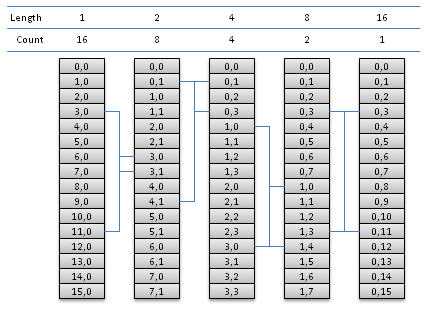# OpenCL Fast Fourier Transform

Eric Bainville - May 2010

As a starting point, we will implement a simple out of place radix-2 where, at each step, all sequences are stored in contiguous entries of the arrays.Radix-2 DFT, N=16. For each iteration, we show the contents (a,k)Length of the current array, a is the start index of the transformed sequence, and k the value index in the DFT. Count is the number of sub-sequences, and Length is their length. For each iteration, an example of radix-2 butterfly is displayed (blue lines). Butterfly inputs are always at distance 8=N/2 in the array, and outputs are at distance Length.

If we run one thread for each butterfly taking indices i and i+N/2 as inputs, all memory read operations will always be coalesced (1 single memory access per group of 16 threads executed at the same time) as soon as N/2 is large enough. Memory write operations will be coalesced for all iterations but the first few.

The kernel operates on buffer x and stores the output in y. The next iteration is then called with (y,x), etc.

Let's first define some useful macros and functions, operating on complex numbers stored as float2:

```// a*b => a, b unchanged
#define MUL(a,b,tmp) { tmp=a; a.x=tmp.x*b.x-tmp.y*b.y; a.y=tmp.x*b.y+tmp.y*b.x; }

// DFT2(a,b) => (a,b)
#define DFT2(a,b,tmp) { tmp=a-b; a+=b; b=tmp; }

// Return cos(alpha)+I*sin(alpha)
float2 exp_alpha(float alpha)
{
float cs,sn;
sn = sincos(alpha,&cs);
return (float2)(cs,sn);
}
```

```// N = size of input, power of 2.
// T = N/2 = number of threads.
// I = index of current thread, in 0..T-1.
// DFT step, input is X[N] and output is Y[N].
// P is the length of input sub-sequences, 1,2,4,...,N/2.
// Cell (S,K) is stored at index S*P+K.
__kernel void fft_radix2(__global const float2 * x,__global float2 * y,int p)
{
int i = get_global_id(0); // number of threads
int t = get_global_size(0); // current thread
int k = i & (p-1); // index in input sequence, in 0..P-1

// Input indices are I and I+T.
float2 u0 = x[i];
float2 u1 = x[i+t];

// Twiddle input (U1 only)
float2 twiddle = pow_theta(k,p);
float2 tmp;
MUL(u1,twiddle,tmp);

// Compute DFT
DFT2(u0,u1,tmp);

// Output indices are J and J+P, where
// J is I with 0 inserted at bit log2(P).
int j = (i<<1) - k; // = ((i-k)<<1)+k
y[j] = u0;
y[j+p] = u1;
}
```

This kernel must be called for p=1, then p=2, etc. until p=N/2. The number of threads at each call is N/2, and the work group size WG doesn't matter since all threads are independent. We need to insert a barrier between two kernel execution, to make sure the output array is updated before being used as input in the next call.

For N=224, this kernel requires 24 iterations. We measure the lowest execution time for all feasible values of the work-group size:

Best time54 ms73 ms
Shortest iteration2.0 ms2.6 ms

The ATI compiler does a much better job when using vector types and functions, and the NVidia compiler handles them equally. We will pack one complex number in a float2: (re(a),im(a)), two complex numbers in a float4: (re(a),im(a),re(b),im(b)), etc. The vector of real parts of x is then x.even, and imaginary parts are x.odd.

Let's define some functions handling these representations:

```// Complex product, multiply vectors of complex numbers

#define MUL_RE(a,b) (a.even*b.even - a.odd*b.odd)
#define MUL_IM(a,b) (a.even*b.odd + a.odd*b.even)

float2 mul_1(float2 a,float2 b)
{ float2 x; x.even = MUL_RE(a,b); x.odd = MUL_IM(a,b); return x; }

float4 mul_2(float4 a,float4 b)
{ float4 x; x.even = MUL_RE(a,b); x.odd = MUL_IM(a,b); return x; }

// Return the DFT2 of the two complex numbers in vector A
float4 dft2_2(float4 a) { return (float4)(a.lo+a.hi,a.lo-a.hi); }

// Return cos(alpha)+I*sin(alpha)  (3 variants)
float2 exp_alpha_1(float alpha)
{
float cs,sn;
// sn = sincos(alpha,&cs);  // sincos
cs = native_cos(alpha); sn = native_sin(alpha);  // native sin+cos
// cs = cos(alpha); sn = sin(alpha); // sin+cos
return (float2)(cs,sn);
}
```

Using these functions, we get the following compact kernel:

```// Radix-2 kernel
__kernel void fft_radix2(__global const float2 * x,__global float2 * y,int p)
{
int i = get_global_id(0); // number of threads
int t = get_global_size(0); // current thread
int k = i & (p-1); // index in input sequence, in 0..P-1
x += i; // input offset
y += (i<<1) - k; // output offset
float4 u = dft2( (float4)(
x,
mul_1(exp_alpha_1(-M_PI*(float)k/(float)p),x[t]) ));
y = u.lo;
y[p] = u.hi;
}
```

We measured the 3 variants to compute (cos(α),sin(&alpha)):